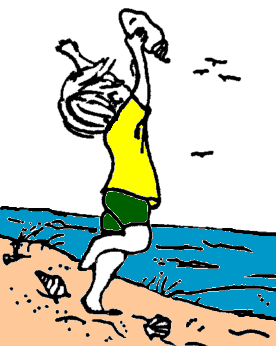Lesson PlansThe lesson plans are divided within each of the Cycles (Applied Science, Universe, Plate Tectonics, Rock, Water, and Life). Each of the cycles is further divided into 4 themes. For example, Applied Science is divided into Science and Math, Physics, Technology, and Build Environment. Each of the themes is divided into the Pre Lab, the Lab, and Post Lab, for each week that you teach it. For example Applied Sciences - Science and Math is taught for 3 weeks. Each week is given an appropriate letter (A is the first week, B is the second). This helps in finding materials and organizing lesson plans.

The Pre Lab introduces the student to the subject matter. Many times the activity relates directly to the Lab, but sometimes it attempts to get the students prepared. We have found that teachers sometimes don’t understand the connection at first, but as they become more familiar with the activities they will comprehend the sequence. Skills are important in completing the lab. Sometimes teachers go directly to the Lab without complete the Pre Lab; this is a mistake. Teachers should also prepare the students for the hands on lab. We have found that anticipation of the subject helps them to remember the lab easily.

When you are doing the first Pre Lab in a cycle, a teacher should inform students that they are moving into a new cycle. They may want to review the last cycle briefly. Review and repeat help students understand and remember large concepts.

The Lab is the portion of the science program that the students tend to remember the most. A hands-on teaching style is the most powerful ways humans learn. The labs are set up for students to work their way through a problem. Students are asked to experiment with a specific objective. They collect data and then make a conclusion on that data. You will find that some students may have the same data and make different conclusions upon their observations. In some experiments there are "no" conclusions, which is a valid way of obtaining scientific information. If something doesn’t work, you know to continue in other directions.

Teachers should talk about five minutes going, over the directions at the beginning. Make sure safety instructions are included. After instructions students should go into their working groups (determined prior to the lab) and begin their activity. In a well-run lab, students should set up and clean up as part of the activity. These skills are important. If a teacher puts down strict rules at the beginning, students will tend to follow them.

The Lab is when parents or volunteers are the most useful. As students are working on the lab sheet, it is suggested that you walk around the room helping individual students. This is the time when you should begin questioning the students about the lab to see they really understand.

Many times the Post Lab is an activity that answers questions that students asked when we were researching the topics for the I. Science MaTe program. We have tried to vary the type of activity that the students are exposed to. Many times there are literature books, art projects, or computer projects. It is important for students to realize that science is all around us.

The Post Lab does not necessarily build on the previous lab, but adds another perspective to the subjects. Many fo the Post Labs can be homework assignments or independent projects.

Approach